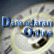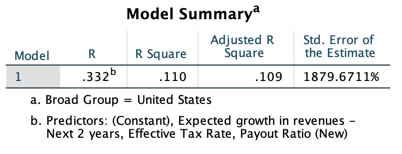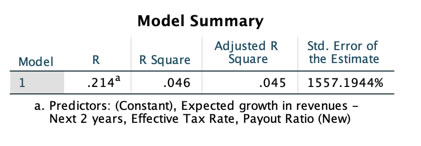##Debt Ratio Regression: January 2019

Variables used in the regression

1. Debt Ratio = Debt/ (Market Value of Equity + Debt): If you can get market value of debt, use it. Else, use book value of debt.
2. Payout Ratio= Dividends/ Net Income, if Net Income is positve, not available if net income is negative.
3. Expected growth rate in EPS- next 5 years= You can use expected or even historical earnings growth, if you don't have an EPS growth forecast
4. Effective Tax Rate = Effective tax rate in most recent year

US Regression## US Regression

#### Debt Ratio = 0.31 - 0.217 Effective Tax Rate - 0.493 Expected Growth rate in EPS +0.007 Payout Ratio

With the following t statistics
Constant: 43.38
Effective Tax Rate: 9.84
Expected Growth Rate in EPS: 12.47
Payout Ratio: 2.25

Global Regression## Global Regression

#### Debt Ratio = 0.32 - 0.107 Effective Tax Rate - 0.441 Expected Growth rate in EPS +0.008 Payout Ratio

With the following t statistics
Constant: 378.49
Effective Tax Rate: 7.43
Expected Growth Rate in EPS: 22.10
Payout Ratio: 4.32

• How do I use this regression?

Assume that you want to estimate the market debt ratio for a firm with the following characteristics, using the Global regression

Payout Ratio = 40%

Effective Tax Rate= 20%

Expected growth rate in EPS = 15%

• Predicted Value

Expected Debt Ratio = 0.32 - .107 (.20) - .441 (.15) +0.008 (.40) = .2357 or 23.57%

If your predicted value is less than zero, your predicted debt ratio is zero.